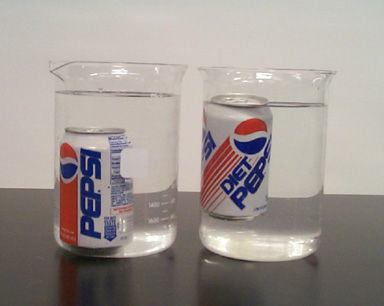Buoyancy of salted waterIn science, buoyancy is an upward force exerted by a fluid that opposes the weight of an immersed object. The above left shows a can of Pepsi sunken in pure water, and the above right shows the same can of Pepsi floating in salted water. Which of the following statements is correct?

a) The buoyancy force upon the right Pepsi can is greater than the buoyancy force upon the left Pepsi can.

b) The gravity force upon the left Pepsi can is greater than the gravity force upon the right Pepsi can.

c) The weights of fluid pushed by the left Pepsi and the right Pepsi cans are the same.

×